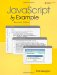# EXERCISES

 1: Create an array of five animals. Use a for loop to display the values stored there. Now add two more animals to the end of the array and sort the array. (Use JavaScript's built-in array methods .) Display the sorted array. 2: Create an associative array called colors . Each element of the array is a string representing the color , e.g., red or blue . Use the for/in loop to view each element of the array with a color of the font the same color as the value of the array element being displayed. 3: Create a function that will return the current month by its full name . Use the Date object to get the current month. Months are returned as 0 for January, 1 for February, 2 for March, etc. Output should resemble: ` ` ` The current month is January. ` Hint: You can create an array, starting at index 0, and assign a month value to it; e.g., month="January" or use a switch statement, e.g., case 0: return "January" . 4: An invoice is due and payable in 90 days. Write a function that will display that date. 5: How many days until your birthday? Write a function to calculate it. 6: To calculate the balance on a loan, the following formula is used: PV = PMT * ( 1 “ (1 + IR ) “NP ) / IR PV is the present value of the loan; PMT is the regular monthly payment of the loan; IR is the loan's interest rate; NP is the number of payments remaining. Write a JavaScript statement to represent this formula. 7: Using the formula to calculate the loan balance from the last exercise, write a function that will calculate the principle balance left on a loan where the monthly payments are \$600, the annual interest rate is 5.5%, and there are 9 years remaining on the loan. Use the toFixed() Number method to format the output. 8: Apply the ceil(), floor() , and round() methods to the number 125.5567 and display the results. 9: Create an array of 10 fortune cookies that will be randomly displayed each time the user reloads the page. 10: Create a string prototype that can be used to create an italic, Verdana font, point size 26. 11: Calculate the circumferance of a circle using the Math object. 12: Write a JavaScript program that uses the Array and Math objects. Create an array of 5 sayings, for example: " A stitch in time saves 9" , or "Too many cooks spoil the broth" . Each time the Web page is visited, print a random saying. 13: Use the Date object to print today's date in this format: ` ` ` Today is Friday, June 16, 2003. ` Calculate and display the number of days until your next birthday. Create a prototype for the Date object that will print the months starting at 1 instead of 0. 14: Create a String object containing "Jose lived in San Jose for many years." Find the index for the second Jose . Get the substring ear from years . Display the string in a blue, italic font, point size 12, all uppercase.JavaScript by Example (2nd Edition)
ISBN: 0137054890
EAN: 2147483647
Year: 2003
Pages: 150
Authors: Ellie Quigley

Similar book on Amazon